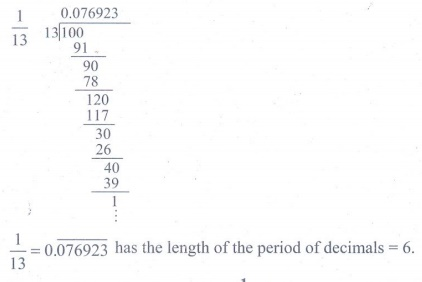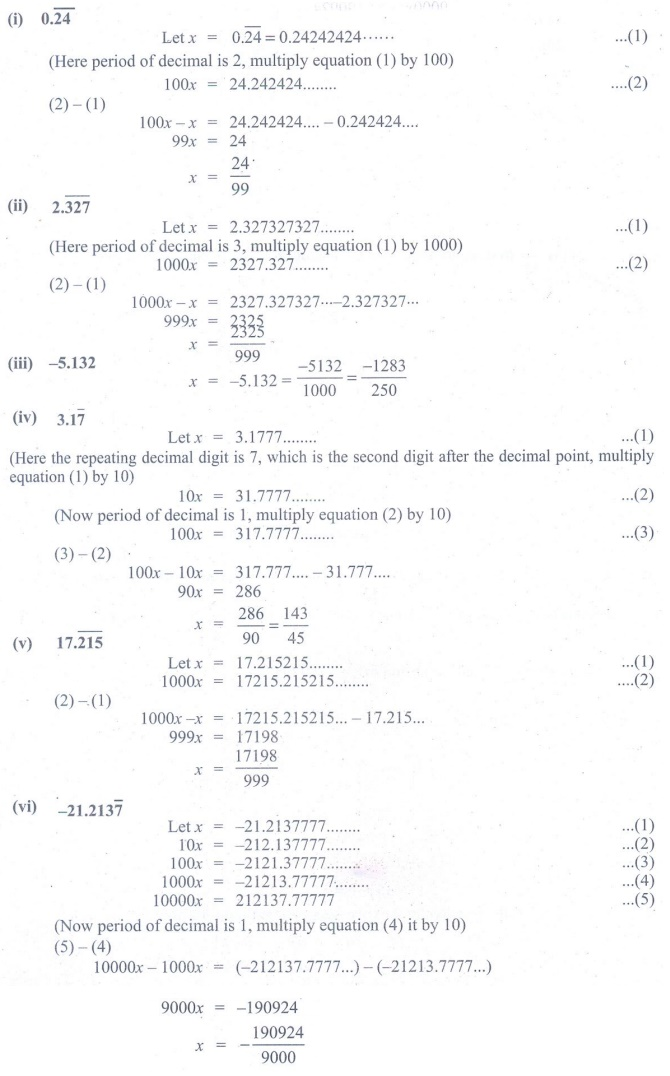Home | | Maths 9th std | Exercise 2.2: Irrational Numbers

# Exercise 2.2: Irrational Numbers

Maths : Real Numbers: Book Back, Exercise, Example Numerical Question with Answers, Solution: Exercise 2.2: Irrational Numbers

Exercise 2.2

1. Express the following rational numbers into decimal and state the kind of decimal expansion

(i) 2/7 (ii) − 5 (3/11) (iii) 22/3 (iv) 327/2002. Express 1/13 in decimal form. Find the length of the period of decimals.3. Express the rational number 1/33 in recurring decimal form by using the recurring decimal expansion of 1/11. Hence write 71/33 in recurring decimal form.4. Express the following decimal expression into rational numbers.5. Without actual division, find which of the following rational numbers have terminating decimal expansion.

(i) 7/128 (ii) 21/15 (iii) 4 (9/35) (iv) 219/2200Tags : Numerical Problems with Answers, Solution | Real Numbers | Maths , 9th Maths : UNIT 2 : Real Numbers
Study Material, Lecturing Notes, Assignment, Reference, Wiki description explanation, brief detail
9th Maths : UNIT 2 : Real Numbers : Exercise 2.2: Irrational Numbers | Numerical Problems with Answers, Solution | Real Numbers | Maths